# KSEEB Solutions for Class 8 Maths Chapter 5 Squares, Square Roots, Cubes, Cube Roots Additional Questions

Students can Download Maths Chapter 5 Squares, Square Roots, Cubes, Cube Roots Additional Questions and Answers, Notes Pdf, KSEEB Solutions for Class 8 Maths helps you to revise the complete Karnataka State Board Syllabus and score more marks in your examinations.

## Karnataka State Syllabus Class 8 Maths Chapter 5 Squares, Square Roots, Cubes, Cube Roots Additional Questions

Question 1.
Match the following numbers ¡n the column A with their squares ¡n the column B.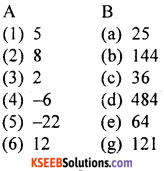Solution:
(1) (a) 25
(2) (e) 64
(3) (f) 4
(4) (c) 36
(5) (d) 484
(6) (b) 144

Question 2.
Choose the correct option:
(a) The number of perfect squares from 1 to 500 is
A. 1
B. 16
C. 22
D. 25
Solution:
C. 22(b) The last digit of a perfect square can never be
A. 1
B. 3
C. 5
D. 9
Solution:
B. 3

(c) If a number ends in 5 zeros, its square ends in
A. 5 zeros
B. 8 zeros
C. lo zeros
D. 12 zeros
Solution:
C. 10 zeros

(d) Which could be the remainder among the following when a perfect square is devided by 8?
A. 1
B. 3
C. 5
D. 7
Solution:
A. 1

(e) The 6th triangular number is
A. 6
B. 10
C. 21
D. 28
Solution:
C. 21Question 3.
Consider all integers froni – 10 to 5 and square each of them. How many distinct numbers you get?
Solution:
(-10)2 = 100, (-9)2 = 81, (-8)2
= 64,(-7)2 = 49
(-6)2 = 36, (5)2 = 25, (-4)2 16, (-3)2 =9,
(2)2 =4, (-1)2 = 1, 02 = 0, 12 = 1, 22 = 4, 32 = 9, 42 = 16, 52 = 25
There are 11 distinct numbers.

Question 4.
Write the digit in units place when the following numbers are squared.
Solution: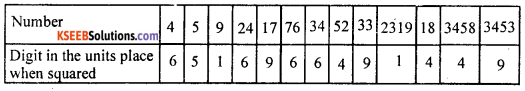Question 5.
Write all the numbers from 400 to 425 which end in 2, 3, 7 or 8. Check if any of these in a perfect square.
Solution:
402, 403, 407, 408, 412, 413, 417, 418, 422, 423
None of these is a perfect square.

Question 6.
Find the sum of the digits of (1111111111)2.
Solution:
Observe the pattern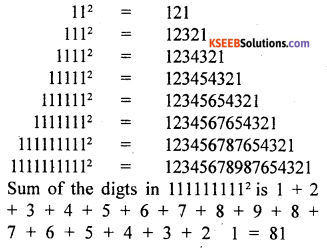Question 7.
Suppose x2 + y2 = Z2
(i) If x = 4 and y = 3 find z
(ii) If x = 5 and z = 13 find y
(iii) If y = 15 and z = 17 find x.
Solution:
(i) x = 4, y = 3
x2 + y2 = z2
42 + 32 = z2
16 + 9 = z2
25 = z2
∴ z = √25 = 5

(ii) x = 5, z = 13
x2 + y2 = z2
52 + y2 = 132
25+ y2 =169
y2 =169 – 25
y2 = 144
y = √144 = 12

(iii) y = 15, z = 17
x2+ y2 = z2
x2 + 152 = 172
x2 + 225 = 289
x2 = 289 – 225
x2 = 64
x = √ 64 = 8Question 8.
A sum of Rs. 2304 is equally distributed among several people. Each gets as many rupees as the number of persons. How much does each one get?
Solution: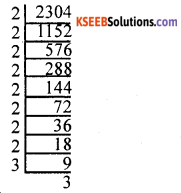Let the number of persons be x. Each person get rupees x.
∴ x × x = 2304
x2 = 2304
x = √2304
2304 = 2 × 2 × 2 × 2 × 2 × 2 × 2 × 3 × 3
= 2 × 2 × 2 × 2 × 2 × 2 × 2 × 2 × 2 × 3
= 16 × 3 × 16 × 3
2304 = 48 × 48
√2304 = 48 Each person gets Rs. 48

Question 9.
Define a new addition e on (be set of all natural numbers by m * n = m2 + n2
(i) Is N closed under e?
(ii) Is * commutative on N?
(iii) Is * associative on N?
(iv) Is there an identity element in N with respect * ?
Solution:
(i) Let m 2 and n 5
m * n = m2 + n2
2 * 5 = 22 + 52 = 4 + 25 = 29 ∈ N
∀ m, n ∈ N, m2 + n2 ∈ N
∴ * is closed under N.

(ii) Let m = 4 and n = 7
m * n = m2 + n2 = 42 + 72 = 16 + 49 = 65
n * m = n2 + m2 = 72 + 42 = 49 + 16 = 65
∴ m * n = n * m ∀ m, n ∈ N
Hence * is commutative on N.(iii) Lei m = 2, n = 3 and p = 4.
m * (n * P) = 2 * (3*4)
= 2*(32 + 42) = 2*(9 + 16)
= 2*25 = 22 + 252 = 4 + 625
= 629 ….(i)
(m * n) * p = (2 * 3)*4
=(22 + 32) * 4 = (4 * 9) * 4
= 13 * 4 = 132 + 42.
= 169 + 16 = 185 …(ii)
From (i) and (ii) m*(n*p) ≠ (m*n)*p
∴ * is not associative on N.

(iv) Let K be the identity element then m * k = m, m2 + k2 = m2 which means
k2 = m2 – m2 = O
K = 0, O does not belong to N.
There is no identity element in N with respect to *.Question 10.
(Exploration) Find all the perfect squares from 1 to 500 each of which is a sum of two perfect squares.
Solution:
Perfect squares from 1 to 500 are 1, 4, 9, 1, 25, 36, 49, 64, 81, 100, 121, 144, 169, 196, 225, 256, 289. 324, 361, 400, 441, 484.
25 = 9 + 16
100 =36 + 64
169 = 25 + 144
289 = 225 + 64

Question 11.
Supposes the area of a square field is 7396 find its perimeter.
Solution:
Let each side of the square be
l2 = 7396 = 2 × 2 × 43 × 43 = 2 × 43 × 2 × 43
l2 =7396 = 86 × 86.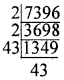∴ √7396 = 86 = l
Perimeter = 4l = 4 × 86 = 344 m.

Question 12.
Can 1010 be written as a difference of two perfect squares?
Solution:
If a2 – b2 = 1010 for any two integers a and b then either both ‘a’ and ‘b’ are odd or both even. Hence a2 – b2 is divisible by 4. But 1010 is not divisible by 4. Hence 1010 is not the difference of two perfect squares.Question 13.
What are the remainders when a perfect cube is divided by 7?
Solution:
When the perfect cubes 8, 27, 64, 125, 216, 343, 512, 729, 1000 are divided by 7 the remainders respectively are 1, 6, 1, 6, 6, 0, 1, 1, 6. Hence the remainders are 0. 1 or 6.

Question 14.
What is the least perfect square which leaves the remainder 1 when divided by 7 as welt as by 11?
Solution:
The least number divisible by both 7 and 11 is 7 × 11 = 77. When 1 is added to 77 we get 78. But 78 is not a perfect square. (77 × 2) + 1 = 154 + 1 = 155 is not a perfect square. In the same way, continue we find that
(77 × 15) + 1 = 1155 + 1 = 1156 in a perfect square.
∴ The number required is 1156 = 342

Question 15.
Find two smallest perfect squares whose product is a perfect cube.
Solution:
4 and 16 are perfect squares. 4 × 16 = 64 is a perfect cube.

Question 16.
Find a proper positive factor of 48 and a proper positive multiple of 48 which add up to a perfect square. Can you prove that there are infinitely many such pairs? By considering 16 as a factor of 48 and 48 as a factor of 240, we can write 16 + 240 = 162.
Solution:
Multiple of 48 is 48 and considering it as 48l where l = m (3m + 2), m – 1, 2, 3 …. we can get infinite numbers.